Journal of Arid Land2020, Vol. 12Issue (5): 865-886    DOI: 10.1007/s40333-020-0075-9
 Research article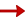Assessing the effects of vegetation and precipitation on soil erosion in the Three-River Headwaters Region of the Qinghai-Tibet Plateau, China
HE Qian1, DAI Xiao'ai1,*(), CHEN Shiqi2
1College of Earth Science, Chengdu University of Technology, Chengdu 610059, China
2College of Foreign Languages and Cultures, Chengdu University of Technology, Chengdu 610059, China
 Download: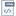HTMLPDF(2546KB) Export: BibTeX | EndNote (RIS)

Abstract

Soil erosion in the Three-River Headwaters Region (TRHR) of the Qinghai-Tibet Plateau in China has a significant impact on local economic development and ecological environment. Vegetation and precipitation are considered to be the main factors for the variation in soil erosion. However, it is a big challenge to analyze the impacts of precipitation and vegetation respectively as well as their combined effects on soil erosion from the pixel scale. To assess the influences of vegetation and precipitation on the variation of soil erosion from 2005 to 2015, we employed the Revised Universal Soil Loss Equation (RUSLE) model to evaluate soil erosion in the TRHR, and then developed a method using the Logarithmic Mean Divisia Index model (LMDI) which can exponentially decompose the influencing factors, to calculate the contribution values of the vegetation cover factor (C factor) and the rainfall erosivity factor (R factor) to the variation of soil erosion from the pixel scale. In general, soil erosion in the TRHR was alleviated from 2005 to 2015, of which about 54.95% of the area where soil erosion decreased was caused by the combined effects of the C factor and the R factor, and 41.31% was caused by the change in the R factor. There were relatively few areas with increased soil erosion modulus, of which 64.10% of the area where soil erosion increased was caused by the change in the C factor, and 23.88% was caused by the combined effects of the C factor and the R factor. Therefore, the combined effects of the C factor and the R factor were regarded as the main driving force for the decrease of soil erosion, while the C factor was the dominant factor for the increase of soil erosion. The area with decreased soil erosion caused by the C factor (12.10×103 km2) was larger than the area with increased soil erosion caused by the C factor (8.30×103 km2), which indicated that vegetation had a positive effect on soil erosion. This study generally put forward a new method for quantitative assessment of the impacts of the influencing factors on soil erosion, and also provided a scientific basis for the regional control of soil erosion.

Received: 23 March 2020      Published: 10 September 2020
Corresponding Authors: Xiao'ai DAI     E-mail: daixiaoa@cdut.cn
About author: *Corresponding author: DAI Xiao'ai (E-mail: daixiaoa@cdut.cn)
 Service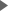E-mail this articleAdd to my bookshelfAdd to citation managerE-mail AlertRSS Articles by authorsQian HEXiao'ai DAIShiqi CHEN
 Cite this article: HE Qian, DAI Xiao'ai, CHEN Shiqi. Assessing the effects of vegetation and precipitation on soil erosion in the Three-River Headwaters Region of the Qinghai-Tibet Plateau, China. Journal of Arid Land, 2020, 12(5): 865-886. URL: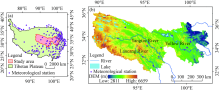Fig. 1 Location of the Three-River Headwaters Region (TRHR) in the Qinghai-Tibet Plateau, China (a) and overview of the TRHR (b). DEM, digital elevation model.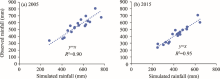Fig. 2 Verification of the annual rainfall interpolation results in 2005 (a) and 2015 (b)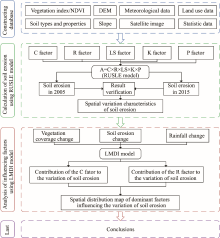Fig. 3 Workflow and methods of the study. DEM, digital elevation model; RUSLE, revised universal soil loss equation; LMDI, logarithmic mean Divisia index; A, the amount of soil erosion; C factor, vegetation cover factor; R factor, rainfall erosivity factor; LS factor, topographic factor; K factor, soil erodibility factor; P factor, support practice factor.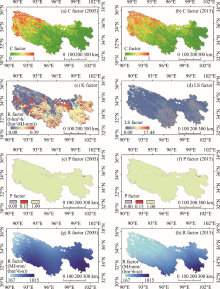Fig. 4 Layers of the RUSEL factors in the TRHR. (a), distribution of C factor values in 2005; (b), distribution of C factor values in 2015; (c), distribution of K factor values; (d), distribution of LS factor values; (e), distribution of P factor values in 2005; (f), distribution of P factor values in 2015; (g), distribution of R factor values in 2005; (h), distribution of R factor values in 2015.Table 1 Criteria for assessing the relative effects of the C factor and R factor on the variation of soil erosion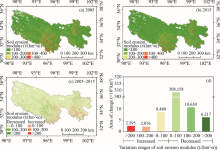Fig. 5 Spatial distribution maps and statistical charts of soil erosion modulus in 2005 and 2015. (a), spatial distribution map of soil erosion modulus in 2005; (b), spatial distribution map of soil erosion modulus in 2015; (c), spatial distribution map of the variation of soil erosion modulus from 2005 to 2015; (d), area of different variation ranges of soil erosion modulus (the horizontal axis represents the different ranges of the variation in soil erosion modulus).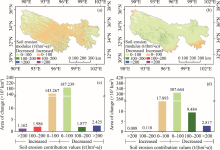Fig. 6 Spatial distribution maps (a and b) and statistics of the areas (c and d) of the contributions of the C factor and the R factor to the variation of soil erosion. (a), spatial distribution map of the contribution of the C factor to the variation of soil erosion; (b), spatial distribution map of the contribution of the R factor to the variation of soil erosion; (c), area chart of the contribution of the C factor to the variation of soil erosion; (d), area chart of the contribution of the R factor to the variation of soil erosion.Fig. 7 Cumulative soil erosion contribution values of the C factor (a) and the R factor (b). (a), contribution values of the NDVI in different changing ranges to the variation of soil erosion from 2005 to 2015; (b), contribution values of the R factor in different changing ranges to the variation of soil erosion from 2005 to 2015. The vertical axes represent soil erosion contribution values of the NDVI change and the R factor change.Fig. 8 Spatial distribution map of dominant factors influencing the decrease of soil erosion. DEC, decreased soil erosion due to the C factor; DER, decreased soil erosion due to the R factor; DECR, decreased soil erosion due to the C factor and the R factor.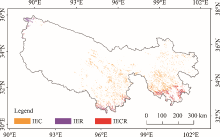Fig. 9 Spatial distribution map of dominant factors influencing the increase of soil erosion. IEC, increased soil erosion due to the C factor; IER, increased soil erosion due to the R factor; IECR, increased soil erosion due to the C factor and the R factor.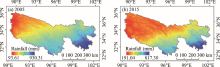Fig. S1 Spatial distribution of total annual rainfall in 2005 (a) and 2015 (b)